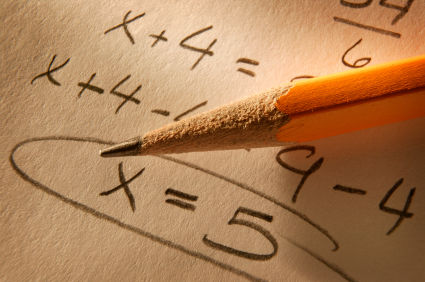# Algebra I Readiness Assessment-timed 2016

50 Questions | Total Attempts: 1661SettingsToday you will be taking an Algebra I Readiness Assessment. Do your best to answer each question. You will have 125 minutes to complete this test. Calculators are NOT permitted for use on this test.

• 1.
• A.

3

• B.

18

• C.

108

• D.

216

• 2.
In trail mix, the ratio of cups of peanuts to chocolate candies is 3 to 2. How many cups of chocolate candies would be needed for 9 cups of peanuts?
• A.

6 cups

• B.

3 cups

• C.

4 cups

• D.

8 cups

• 3.
• A.

4

• B.

24

• C.

98

• D.

7

• 4.
If hamburger meat costs \$2.25 per pound, how much does 0.8 pound of hamburger cost?
• A.

\$2.81

• B.

\$1.13

• C.

\$1.80

• D.

\$2.13

• 5.
What is the ratio of side AB to side BC, in simplest form?
• A.

1:1

• B.

2:3

• C.

3:2

• D.

4:3

• 6.
Which of the following has a unit rate of 17 miles per hour?
• A.

60 miles in 2 hours

• B.

85 miles in 5 hours

• C.

90 miles in 10 hours

• D.

120 miles in 15 hours

• 7.
Which expression is NOT equivalent to the others?
• A.

4(m + 2)

• B.

3m + 8 + m

• C.

4m + 4

• D.

2(1 + m) + m + 6 + m

• 8.
Which statement is true?
• A.

0.75

• B.

6.12>6.16

• C.
• D.
• 9.
• A.

3 gallons

• B.

12 gallons

• C.

2 gallons

• D.
• 10.
For birthday parties, the skating rink charges \$100 to reserve the rink and then \$5 per person. Write an expression to represent the cost for any number of people.
• A.

100 – 5n

• B.

100 + 5n

• C.

5(n + 100)

• D.

5(n – 100)

• 11.
The seventh grade class is going on a trip to the state fair. The trip costs \$52. Included in that price is \$11 for a concert ticket and the cost of two passes, one for rides and one for game booths. Each of the passes cost the same price. Write an equation representing the cost of the trip and determine the price of one pass.
• A.

2x – 11 = 52; \$31.50

• B.

3x = 52; \$17.33

• C.

2x = 52; \$26

• D.

52 = 11 + 2x; \$20.50

• 12.
• A.
• B.
• C.
• D.
• 13.
In the picture of the three triangles, two are similar. Which statement below is true?
• A.
• B.
• C.
• D.
• 14.
A rectangle has vertices P(1, 0), Q(6, 0), R(6, 6), and S(1, 6).What is the area of rectangle PQRS?
• A.
• B.
• C.
• D.
• 15.
Find the circumference.
• A.
• B.
• C.
• D.
• 16.
What is the area of a triangle with a height of 20 meters and a base of 16 meters?
• A.
• B.
• C.
• D.
• 17.
Determine the surface area of rectangular prism with height 5 inches, width 7 inches, and length 12 inches.
• A.
• B.
• C.
• D.
• 18.
The volume of a cube is  .  What is the edge length?
• A.

4 in.

• B.

in.

• C.

in.

• D.

8 in.

• 19.
If a rectangle, measuring 2 in. by 7 in., is enlarged by using a scale factor of 2, what will the area be of the new rectangle?
• A.

56

• B.

28

• C.

14

• D.

98

• 20.
Solve the following inequality. -0.5- 5  -1.5
• A.

X   -7

• B.

X   7

• C.

X   7

• D.

X   -7

• 21.
Find the approximation of .
• A.

6.0

• B.

6.2

• C.

7.0

• D.

7.5

• 22.
Which equation corresponds to the statements “the length L  of the rectangle is four less than twice the width W” ?
• A.

W = 4 - 2L

• B.

W = 4L - 2

• C.

L= 2W - 4

• D.

L = 4 - 2W

• 23.
Simplify
• A.
• B.
• C.
• D.
• 24.
Evaluate 5(n + 3) - 7n if n=.
• A.

20

• B.

7.5

• C.

6

• D.

14

• 25.
Divide .
• A.
• B.
• C.
• D.
Related Topics